# Class 9 RD Sharma Solutions – Chapter 1 Number System – Exercise 1.2

• Last Updated : 03 Mar, 2021

### iii) 15/4

Solutions:

(i) 42/100

Attention reader! All those who say programming isn't for kids, just haven't met the right mentors yet. Join the  Demo Class for First Step to Coding Coursespecifically designed for students of class 8 to 12.

The students will get to learn more about the world of programming in these free classes which will definitely help them in making a wise career choice in the future.

Using long division method: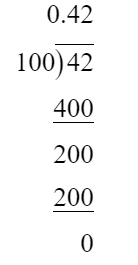So, 42/100 = 0.42

(ii) 327/500

Using long division method: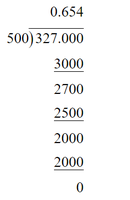So, 327/500 = 0.654

(iii) 15/4

Using long division method: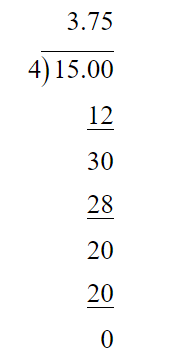So, 15/4 = 3.75

### (vi) 33/26

Solutions:

(i) Using long division method: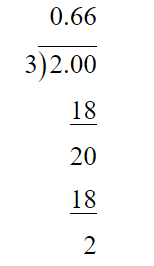So, 2/3 = 0.66.. =(ii) Using long division method: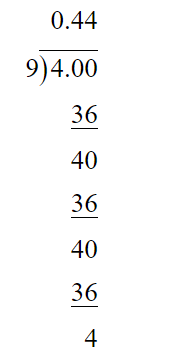So, -4/9 = -0.44.. =(iii) Using long division method: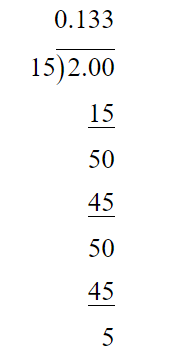So, -2/15 = (- 0.133..) =(iv) -22/13 = -(1 + 9/13)

Using long division method: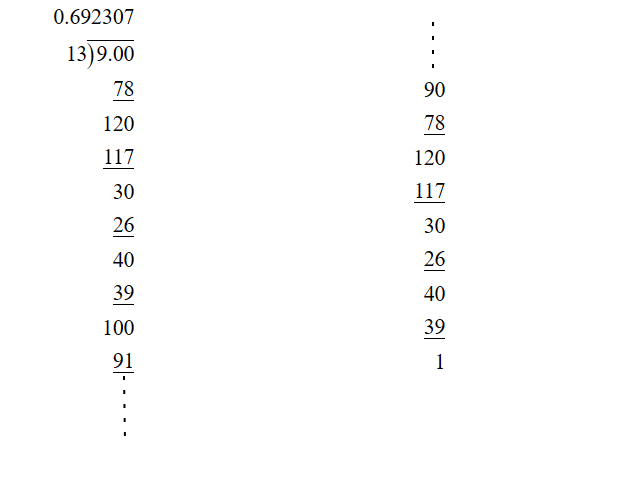So, -22/3 = -(1.692307…) =(v) 437/999

Using long division method: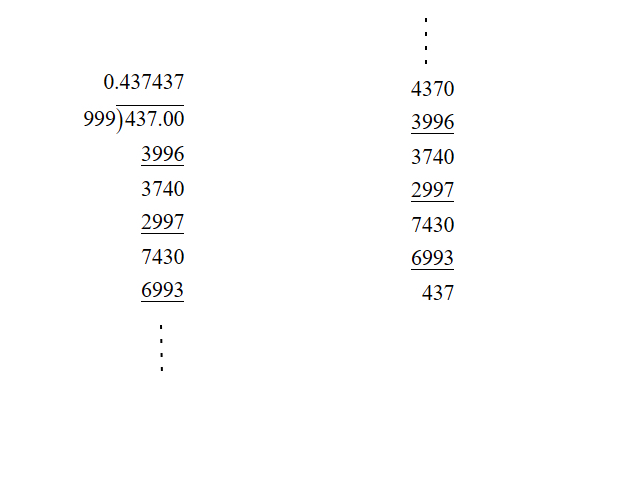So, 437/999 = 0.437… =(vi) 33/26 =Using long division method: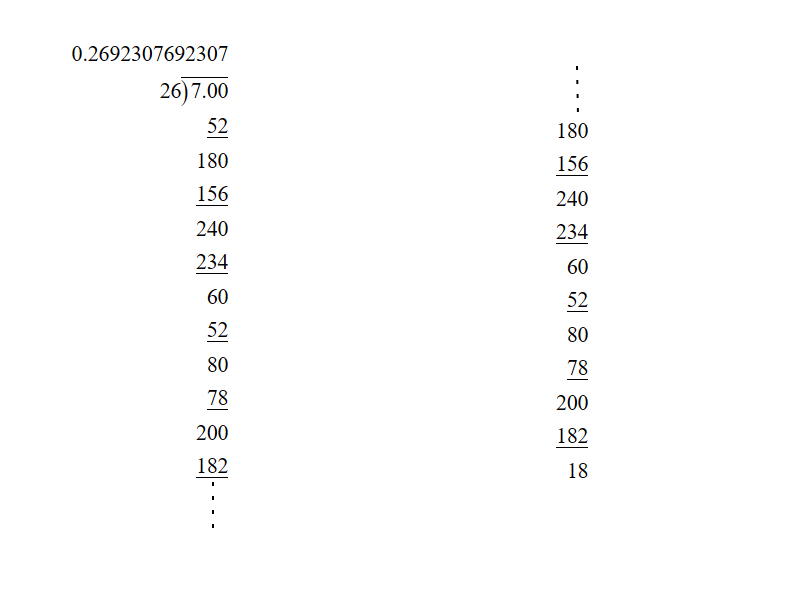So, 33/26 = 1.2692307… =### Question 3. Look at several examples of rational numbers in the form p/q (q ≠ 0), where p and q are integers with no common factors other than 1 and having terminating decimal representations. Can you guess what property q must satisfy?

Solution:

p/q will be terminating decimal when prime factorization of q must have factors 2 or 5 or both.

My Personal Notes arrow_drop_up